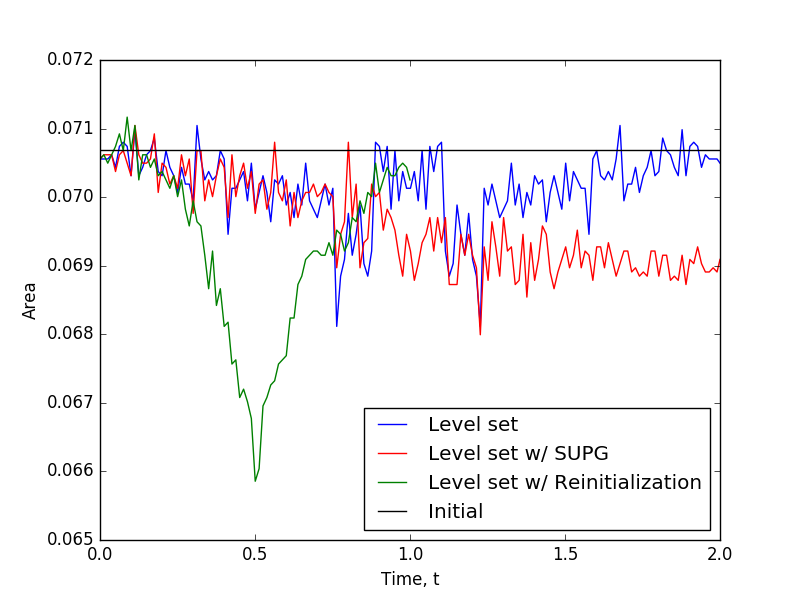# Vortex

A classic and challenging benchmark problem for the level set is the vortex problem. This problem is similar to the rotating bubble (see Rotating Bubble Example). The problem involves initializing (see Theory) with a "bubble" of radius 0.15 at for . This bubble is then advected with the a vortex velocity field (see LevelSetOlssonVortex), so that, at , the bubble should return to its original position.

The only significant difference between the Rotating Bubble Example and this example is the velocity function; therefore, a detailed discussion of the varying input files is omitted.

As is the case for the the Rotating Bubble Example three scenarios are considered: the level set equation alone, with SUPG stabilization, and with reinitialization. The complete input files are located in the modules/level_set/examples/vortex directory of MOOSE.

Figures Figure 1 through Figure 3 show the results of the three scenarios. The solution quality of the reinitialization case (Figure Figure 3) is obviously lacking. The reasoning the solution is behaving in the manner is not fully understood, with respect to the level set module. This is an something that needs to be investigated further.Figure 1: Results from solving the vortex problem with the level set equation alone.Figure 2: Results from solving the vortex problem with the level set equation using SUPG stabilization.Figure 3: Results from solving the vortex problem with the level set equation with reinitialization.

The area comparison of the three methods is shown in Figure Figure 4, again the reinitialization example is not performing as expected. The area results shown here indicates that reinitialization step as implemented in this module may be lacking. Additional work should be done to add additional stabilization and reinitialization schemes and uncover the inconsistencies seen here.Figure 4: Comparison of area inside the vortex during simulations.